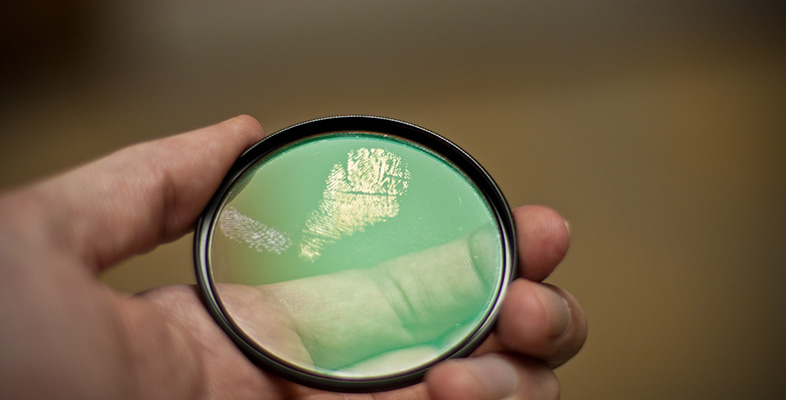Forensic science and fingerprints

Start this free course now. Just create an account and sign in. Enrol and complete the course for a free statement of participation or digital badge if available.

Free course

# 3.3 Chemical tests

As well as using the interactions of radiation with matter to help in the identification of materials, forensic scientists make extensive use of chemical tests. Chemical reagents are used to produce a chemical change that results in a change of colour, or some other detectable change. A simple test that can give an indication of the presence of a substance is called a presumptive test. Presumptive tests are often the first kind of test used in looking for a substance but they do not give definite results of the type needed in court.

If you have ever collected wild, field or horse mushrooms you may know a simple colour test. The poisonous mushroom Agaricus xanthodermus can look like a small edible horse mushroom. If in doubt about whether it is an edible variety, mushroom hunters cut the base of the stem or look for the colour when they pull it from the ground. The cut or damaged stem of Agaricus turns a bright chrome-yellow (Figure 9). The yellow stain is formed by a chemical reaction between a substance in the mushroom and the oxygen in the air. Field and horse mushrooms do not show a yellow colour when the stems are cut or damaged.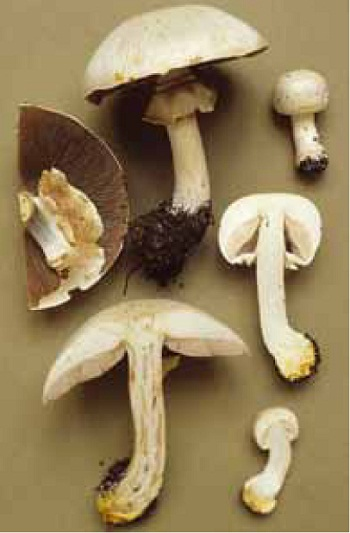Figure 9 The yellow stain on the stems of Agaricus xanthodermus damaged as they are pulled from the ground.

Box 1 briefly summarises some basic chemical concepts with which you should already be familiar. Read through it quickly and check that you can answer the questions correctly.

## Box 1 Some basic chemistry revised

### Atoms

Atoms are the building blocks of all chemical substances. An atom contains three components: negatively charged electrons surrounding a positively charged nucleus containing neutrons and positively charged protons. The different types of atom are called chemical elements and each element has its own symbol.

#### Question 11

What are the names of the elements represented by the following symbols: Ag, Al, C, Cl, Cu, Fe, H, N, Na, O, S?

They are, in order: silver, Ag; aluminium, Al; carbon, C; chlorine, Cl; copper, Cu; iron, Fe; hydrogen, H; nitrogen, N; sodium, Na; oxygen, O; and sulfur, S.

### Molecules: molecular and structural formulas

Molecules are electrically neutral collections of atoms bound together in specific proportions. Each molecule has a particular geometric arrangement of atoms. One way of describing molecules is to use their molecular formula, such as the familiar H2O for a water molecule. The geometry of molecules can be shown approximately in a structural formula. In water the three atoms are held together by two single bonds (Figure 10). A single bond is composed of two electrons shared between two atoms, holding them together.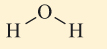Figure 10 The structural formula of water.

### Ions

Molecules are neutral. Ions are charged. The source of all positive ions is the nucleus of an atom which contains one or more protons, each proton in the nucleus having a single positive charge. The nucleus of a hydrogen atom is a single proton, the hydrogen ion, H+. Negative ions are formed when one or more electrons are added to neutral atoms or molecules. Neutral atoms and molecules have exactly equal numbers of protons and electrons. The electrical charge of an electron is exactly equal in magnitude and is opposite in sign to that of a proton, so in terms of electrical charge an electron neutralises a proton.

### Balancing equations

A chemical equation describes a chemical change. A chemical equation has on the left-hand side the formulas of the molecules, atoms or ions that are changed during the chemical reaction - the reactants - and has on the right-hand side the formulas of the new molecules, ions or atoms formed by the chemical change - the products. A balanced equation is one in which there are the same number of atoms of each type on each side of the equation. If there are charges involved, the number of charges also needs to be the same on each side.

#### Question 12

For the three reactions below, which of the equations are balanced chemical equations?

•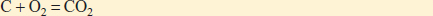Equation label: (Equation 1)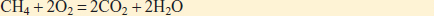Equation label: (Equation 2)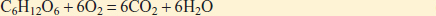Equation label: (Equation 3)

Equations 1 and 3 are balanced, having equal numbers of C, H and O atoms on each side. Equation 2 with one carbon atom on the left-hand side and two carbon atoms on the right-hand side is not balanced. There are also too many oxygen atoms on the right-hand side of Equation 2. The removal of the 2 in front of the CO2 will balance the equation.

Two types of chemical change are particularly important in diagnostic tests; these are acid-base reactions and redox reactions.